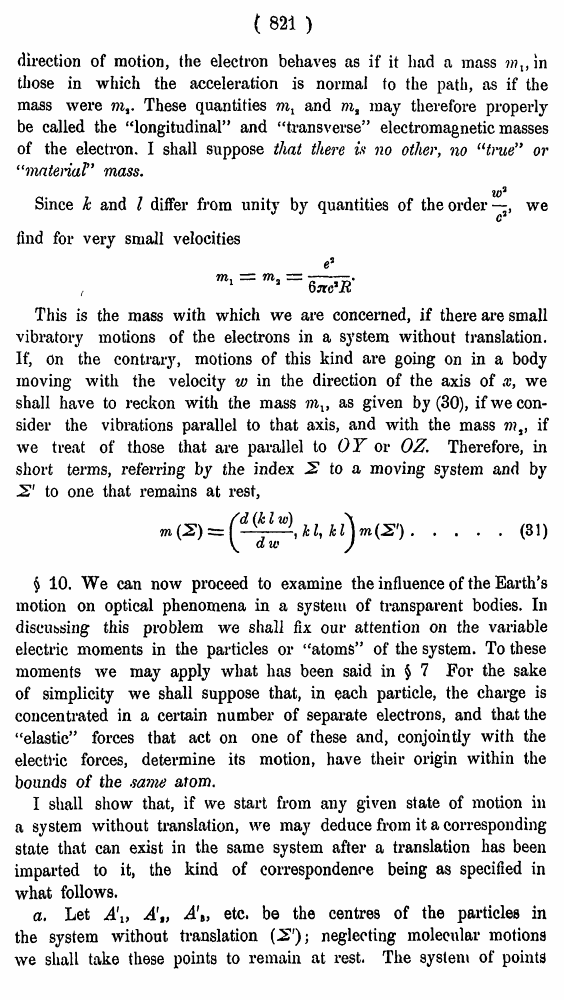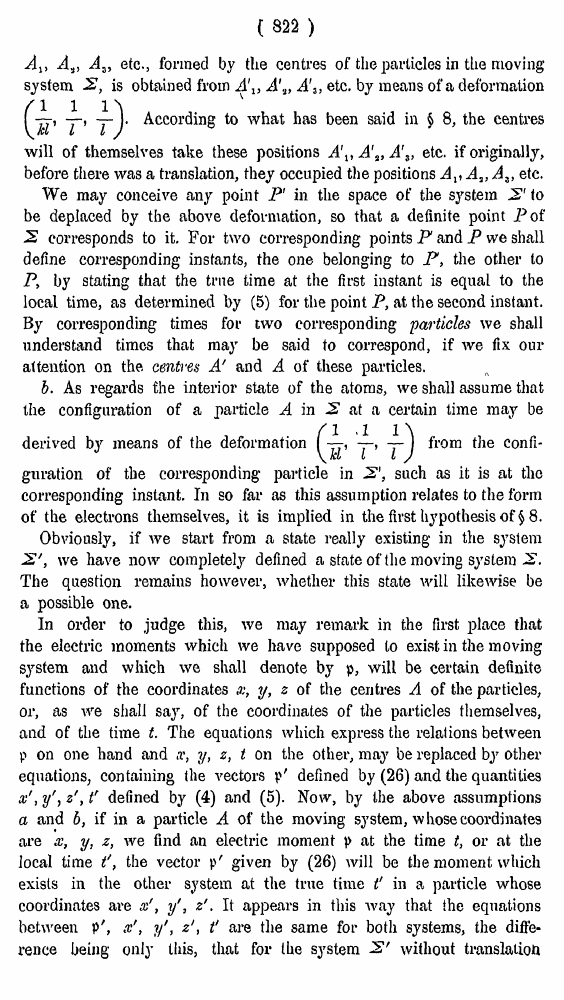# H. A. Lorentz. Electromagnetic phenomena in a system moving with any velocity smaller than that of light. // Proceedings Royal Acad., Amsterdam. Vol. VI., 1904

В начало   Другие форматы   <<<     Страница 821   >>>809  810  811  812  813  814  815  816  817  818  819  820  821 822  823  824  825  826  827  828  829  830  831 ( 821 ) direction of motion, the electron behaves as if it had a mass ???,, in those in which the acceleration is normal to the path, as if the mass were mf. These quantities ml and m% may therefore properly be called the “longitudinal” and “transverse” electromagnetic masses of the electron. I shall suppose that there is no other, no “true” or “material” mass. Since k and I differ from unity by quantities of theordeiwe find for very small velocities ( This is the mass with which we are concerned, if there are small vibratory motions of the electrons in a system without translation. If, On the contrary, motions of this kind are going on in a body moving with the velocity w in the direction of the axis of x, we shall have to reckon with the mass as given by (30), if we consider the vibrations parallel to that axis, and with the mass mt, if we treat of those that are parallel to OY or OZ, Therefore, in short terms, referring by the index 2 to a moving system and by S' to one that remains at rest, .... (31) § 10. We can now proceed to examine the influence of the Earth’s motion on optical phenomena in a system of transparent bodies. In discussing this problem we shall fix our attention on the variable electric moments in the particles or “atoms” of the system. To these moments we may apply what has been said in \$ 7 For the sake of simplicity we shall suppose that, in each particle, the charge is concentrated in a certain number of separate electrons, and that the “elastic” forces that act on one of these and, conjointly with the electlic forces, determine its motion, have their origin within the bounds of the same atom. I shall show that, if we start from any given state of motion in a system without translation, we may deduce from it a corresponding state that can exist in the same system after a translation has been imparted to it, the kind of correspondence being as specified in what follows. a. Let Ai, A'„ A\, etc. be the centres of the particles in the system without translation (2'); neglecting molecular motions we shall take these points to remain at rest. The system of points ( 822 ) A%, A.}, Az, etc., formed by the centres of the particles in the moving system 2, is obtained from A'1} A\, A'3, etc. by means of a deformation According to what has been said in § 8, the centres will of themselves take these positions A\t A's, A'3> etc. if originally, before there was a translation, they occupied the positions Av A,, At> etc. We may conceive any point P' in the space of the system 2' to be deplaced by the above deformation, so that a definite point Pof 2 corresponds to it. For two corresponding points P and P we shall define corresponding instants, the one belonging to P, the other to P, by stating that the true time at the first instant is equal to the local time, as determined by (5) for the point P, at the second instant. By corresponding times for two corresponding 'particles we shall understand times that may be said to correspond, if we fix our attention on the. centres A' and A of these particles. b. As regards the interior state of the atoms, we shall assume that the configuration of a particle A in 2 at a certain time may be derived by means of the deformationfrom the confi guration of the corresponding particle in 2', such as it is at tlio corresponding instant. In so far as this assumption relates to the form of the electrons themselves, it is implied in the first hypothesis of \$8. Obviously, if we start from a state really existing in the system 2', we have now completely defined a state of the moving system 2. The question remains however, whether this state will likewise be si possible one. In order to judge this, we may remark in the first place that the electric moments which we have supposed to exist in the moving system and which we shall denote by p, will be certain definite Functions of the coordinates x, y, z of the centres A of the particles, )r, as we shall say, of the coordinates of the particles themselves, xnd of the time t. The equations which express the relations between ? on one hand and x, ?/, z, t on the other, may be replaced by other jquations, containing the vectors p' defined by (26) and the quantities v', y'j z', t' defined by (4) and (5). Now, by the above assumptions i and b, if in a particle A of the moving system, whose coordinates ire x, y, z, we find an electric moment |> at the time t, or at the ocal time t', the vector p' given by (26) will be the moment which ixists in the other system at the true time t' in a particle whose loordinates are x', yf, z'. It appears in this way that the equations letween p', x', y', zt' are the same for both systems, the diffe* •ence being only this, that for the system 2' without translation